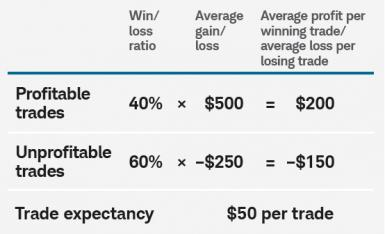Posted on

New All In One Gap Scan + Columns

Today I’ve released updated versions of the All-In-One Gap Scan and the % and \$ Gap Columns.

The improvements to the columns were just to fix a small error I found. But for the scan, several big improvements have been implemented …

1. Choose both min/max gap % size
2. Choose both min/max gap \$ size
3. Choose whether you only want to scan for gaps that haven’t filled yet
4. Choose whether the gap needs to be “significant” — i.e., gapping above the prior high, or below the prior low (completely outside the range of the prior day’s candle)
5. Choose whether the gap needs to be a “pro” or “tier 1” or “shock” gap — i.e., gapping over a prior day red candle, or gapping under a prior day green candle, adding “shock value” to the gap

This single all-in-one scan works during the extended hours / premarket / aftermarket, and continues working as the regular trading hours begin, so you only need to use one scan all day long.

The other change is that the \$ and % values are now all calculated from the prior close instead of the high/low, just for consistency, and whether the gap is over/under the prior day’s candle is specified as a separate criterion in settings, which can be turned on/off at will. This will help keep things consistent and logical no matter what type of trading you do.

Posted on

Trade Expectancy Formula: How to Make Consistent ProfitsThe trade expectancy formula is a super important concept for you to grasp before dumping too much money into the trading world. In any kind of trading there are essentially two forces at work: probability, and risk/reward, and people often misuse the terms. I’ve seen people refer to a their strategy as being “high probability” while their actual win rate is really low. So let’s clear this up once and for all:

Probability = how likely it is that your trade will be correct (Example: 50% as in a coin toss)

Risk/Reward = what you risk on the trade divided by what you stand to gain on the trade (Example: risk \$1 to make \$2 = R/R of 1/2)

Together, these two forces form the “trader’s equation” which is the trade expectancy formula:

(Potential Reward x Win Probability) – (Potential Loss x Loss Probability)

This formula can be used to analyze both individual trades you’re considering taking, and the performance of trading systems in general. The trading system version looks like this:

(Average Win x Win Rate) – (Average Loss x Loss Rate)

In either case, if this number is positive, it’s good. If not, it’s bad.

So in order to take objectively rational trades, you need to make sure every trade you take makes sense in terms of this formula. And you need to occasionally review the ongoing performance of your system by making sure your expectancy remains positive as new trades are added to your trading journal.

In most trading situations you will find the probability of the stock going in your direction hovers around 50%. Author and price action trader Al Brooks says that you should never expect the probability to be any more than 60% or less than 40%, otherwise nobody will be willing to take the other side of the trade. So you should assume the probabilities don’t vary a whole lot in most circumstances.

So what you should actually focus on is looking for asymmetric risk/reward situations. In other words, make sure that you stay out of trades that have a bad risk/reward ratio, and only consider placing trades that have an attractive risk:reward ratio to their potential target.

For instance, if you always look for pullback trades that have at least a 1:2 risk-reward ratio to the prior high, and assume a 50% probability of success, then your trade expectancy formula will look like this:

(2 x 50%) – (1 x 50%)

= 1 – 0.5

= 0.5 expectancy

If you assume a 60% probability, and a 1:2 risk/reward:

(2 x 60%) – (1 x 40%)

= 1.2 – 0.4

= 0.8 expectancy

So higher probability definitely helps. However, if you keep the probability the same as in the first example, and just increase your reward/risk ratio to 3:

(3 x 50%) – (1 x 50%)

= 1.5 – 0.5

= 1.0 expectancy

So risk/reward plays a big role. Also note what the trade expectancy formula looks like for losing system at 40% and 1:1 risk/reward:

(1 x 40%) – (1 x 60%)

= 0.4 – 0.6

= -\$0.2 expectancy

The higher the probability of trades you take, the better. But the primary thing you have control over is lowering your risk/reward (or increasing your reward/risk). This can take several forms, but most practically speaking it probably means cutting your losses and letting your winners run!

In dollar terms that you would probably be trading, here’s what it would look like risking \$500 on each trade with the aim of making \$1000 in profit at a 50% probability of success:

(\$1000 x 50%) – (\$500 x 50%)

= \$500 – \$250

= \$250 expectancy

So in closing, here is a quick reference graph showing which kinds of systems can work and which can’t work based on the trade expectancy formula: